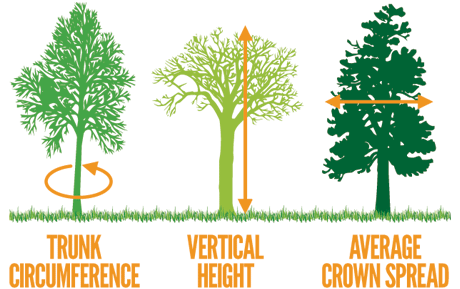Fire season dates: October 1 – December 31
Burning is prohibited from 7 a.m. to 5 p.m. during this time

# Big Tree Program How to Measure a Big Tree

## Big Tree Measurement Guidelines

If you are curious about your own trees, think you know of another that should be in this register or just want to learn how to measure trees, follow the directions below to see if your Big Tree measures up to the competition.

Three tree dimensions are measured to determine their relative size or “bigness.”### Circumference

Circumference is the distance in inches around the trunk of a tree and is usually measured at 41/2’ above average ground level. This is known as Circumference at Breast Height or CBH.

Some considerations when determining CBH:
On sloping ground measure the 4½’ from mid-slope. i.e. “where the acorn grew.”
If a tree forks or a limb protrudes at 4½’ and there is a swell in the circumference, measure the smallest circumference below 4½’. Record this distance above mid-slope.
For trees that fork below 4 ½’ the smallest circumference below the fork is measured and the distance above mid-slope recorded.
For trees that have multiple stems near ground level only the largest stem is measured. For trees that the stems have grown or fused together the CBH measurement indicated as a multi-stem (MS) tree.
On leaning trees the 4½’ is measured along the axis of the trunk, while making sure the circumference is taken at a right angle (perpendicular) to the axis of the trunk.

### Total Height

Height (HT) is the vertical distance measured in feet between the highest twig and the base of the trunk at mid-slope. Dead limbs can be included. The best tools to use for this are the Abney hand level, a hypsometer or a transit. If you do not have those, you can use a straight stick in the following manner.

1. Hold the stick at arm’s length, vertically by its base. The length above your hand should equal the distance from your hand to your eye.
2. Walk backward away from the tree level with the tree’s base. Stop when the part above your hand appears to be the same length as the tree.
3. Sight over your hand to the tree’s base. Remaining motionless, sight over the top of the stick to the top of the tree.
4. Measure how far you are from the tree and you will have the tree’s height in inches.

Crown Spread (CS) is the distance in feet across the outermost leaves/twigs of the crown. To determine crown spread make two measurements: one at the widest spread and a second at a ninety degree angle to the first measurement. Add the two measurements together and divide by two. Dead limbs may be included in measurement.

### Total Points

Circumference (inches) + Total Height (feet) + 1/4 Crown Spread (feet) = Big Tree Total Points.